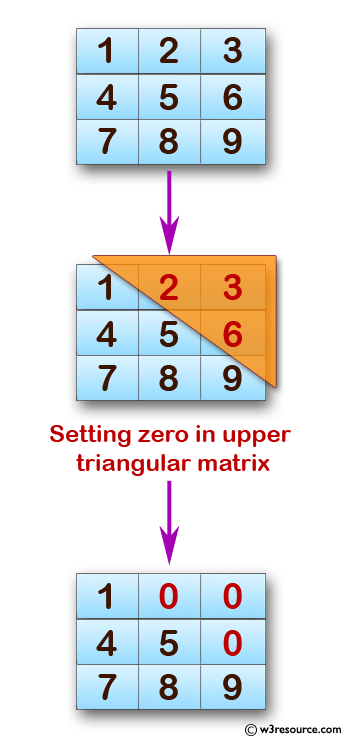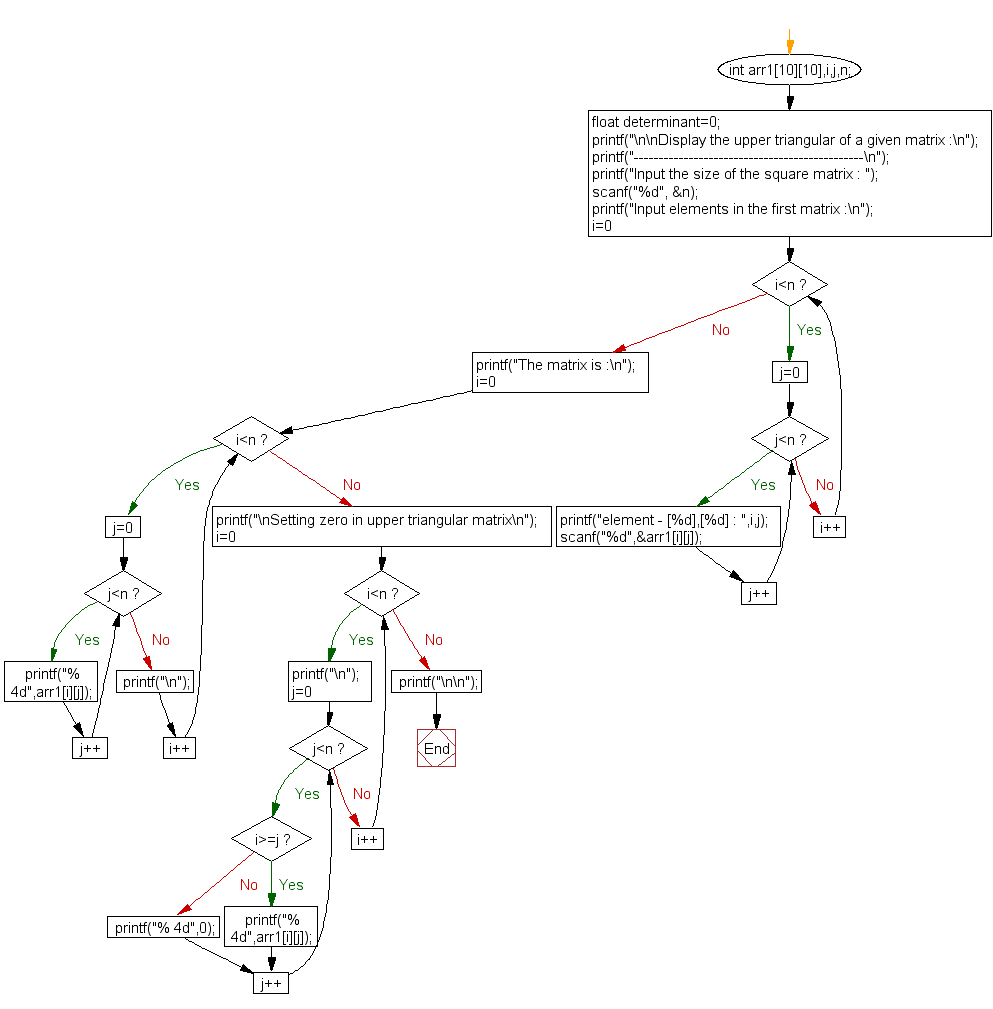﻿ C exercises: Display the upper triangular of a given matrix - w3resource# C Exercises: Display the upper triangular of a given matrix

## C Array: Exercise-27 with Solution

Write a program in C to print or display upper triangular matrix.

Pictorial Presentation:Sample Solution:

C Code:

``````#include <stdio.h>

void main()
{
int arr1,i,j,n;
float determinant=0;

printf("\n\nDisplay the upper triangular of a given matrix :\n");
printf("----------------------------------------------\n");

printf("Input the size of the square matrix : ");
scanf("%d", &n);
printf("Input elements in the first matrix :\n");
for(i=0;i<n;i++)
{
for(j=0;j<n;j++)
{
printf("element - [%d],[%d] : ",i,j);
scanf("%d",&arr1[i][j]);
}
}
printf("The matrix is :\n");
for(i=0;i<n;i++)
{
for(j=0;j<n ;j++)
printf("% 4d",arr1[i][j]);
printf("\n");
}

printf("\nSetting zero in upper triangular matrix\n");
for(i=0;i<n;i++)
{
printf("\n");
for(j=0;j<n;j++)
if(i>=j)
printf("% 4d",arr1[i][j]);
else
printf("% 4d",0);
}
printf("\n\n");
}
```
```

Sample Output:

```Display the upper triangular of a given matrix :
----------------------------------------------
Input the size of the square matrix : 3
Input elements in the first matrix :
element - , : 1
element - , : 2
element - , : 3
element - , : 4
element - , : 5
element - , : 6
element - , : 7
element - , : 8
element - , : 9
The matrix is :
1   2   3
4   5   6
7   8   9
Setting zero in upper triangular matrix

1   0   0
4   5   0
7   8   9
```

Flowchart:C Programming Code Editor:

Improve this sample solution and post your code through Disqus.

What is the difficulty level of this exercise?

﻿